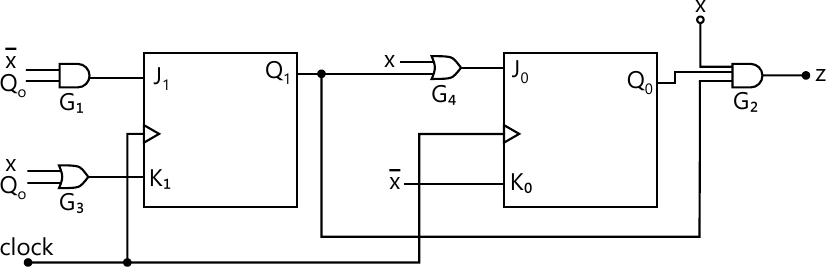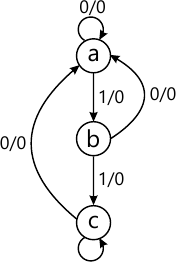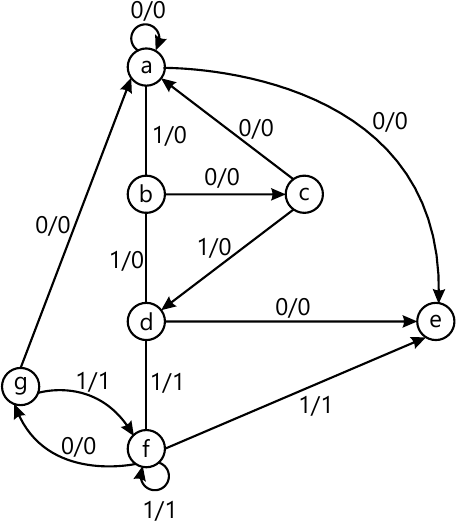MORE IN Design of Programming with Logic
VTU Computer Science (Semester 3)
Design of Programming with Logic
June 2014
Total marks: --
Total time: --
INSTRUCTIONS
(1) Assume appropriate data and state your reasons
(2) Marks are given to the right of every question
(3) Draw neat diagrams wherever necessary

1 (a) Define rise time, fall time in a digital waveform. What is the value of highly duty cycle (duty cycle H) if the frequency of a digital waveform is 5 MHz and the width of the positive pulse is 0.05μs?
4 M
1 (b) Realize the basic gates using only NAND gates.
6 M
1 (c) What is positive and negative logic? List the equivalences in positive and negative logic.
4 M
1 (d) Write a verilog HDL code using structural model for two input AND gate and prepare test-bench to simulate the circuit. Draw the timing diagram generated by simulating the verilog code. Assume 20 ns holding time of each input conbination.
6 M

2 (a) Simplify the Boolean function F(A,B,C,D)=∑m(1,3,5,7,8,10,12,14) by using Karnaugh map method and realize the logic circuit using only NAND gates,
6 M
2 (b) Draw Karnaugh map Y=F(A,B,C,D)=Πm(0,1,2,4,5,10)⋅ d(8,9,11,12,13,15) and get the simplified POS form of K-map.
4 M
2 (c) Get simplified expression of Y=F(A,B,C,D)=∑m(2,3,7,9,11,13)⋅d(1,10,15) using Quine-McClusky method.
10 M

3 (a) What is multiplexer? Design a 4-to-1 multiplexer using logic gates, write the truth table and explain its working principle.
6 M
3 (b) Describe the working principle of 3:8 decoder. Design a circuit that relizes the following functions using a 3:8 decoder and multi-input OR gates.
F1(A, B, C)=∑m(1,3,7)
F2(A, B, C)-∑m(2,3,5)
6 M
3 (c) What is magnitude comparator? Design one bit comparator and write the truth table, logic circuit using basic gates,
6 M
3 (d) How does Programmable Logic Arrays (PLA) differ from a Programmable Array Logic (PAL)?
2 M

4 (a) With the help of neat diagram, explain the working of edge triggered JK flip-flop. Write the state diagram and excitation table.
6 M
4 (b) What is switch contact bounce? Explain the working principle of a simple RS latch debounce circuit.
4 M
4 (c) Write the state table and state diagram for the circuit shown in Fig.Q4(c).10 M

5 (a) What is a shift register? Draw the logic diagram of a 4 bit serial in serial out (SISO) shift register using negative edge triggered JK or D flip-flop and explain its operation with the waveform to shift the binary number 1010 into the register,
8 M
5 (b) Explain with logic diagram the use of 8-bit SISO shift register in serial addition of two 8-bit numbers.
8 M
5 (c) Write verilog HDL code for 4-bit SIPO shift register when all the flip-flop outputs are available externally.
4 M

6 (a) What are asynchronous and synchronous counters? With a neat block diagram, output waveform and truth table, explain a 3-bit binary ripple counter constructed using negative edge triggered JK flip-flops.
10 M
6 (b) Design a mod-5 counter using JK flip-flops having the feature that if an unused state appears, the counter will reset to 000 at the next clock pulse.
10 M

7 (a) With neat block diagrams compare Mcaly model and Moore model of sequential logic system.
4 M
7 (b) Draw the ASM chart for the Mcaly machine shown in fig.Q7(b).8 M
7 (c) Using row elimination method reduce the state diagram shown in fig.Q7(c).8 M

8 (a) What is the binary ladder? Explain the binary ladder with a digital input of 1000.
6 M
8 (b) Defne Accuracy and Resolution with respect to DAC.
4 M
8 (c) With a neat circuit diagram, explain parallel ADC.
10 M

More question papers from Design of Programming with Logic